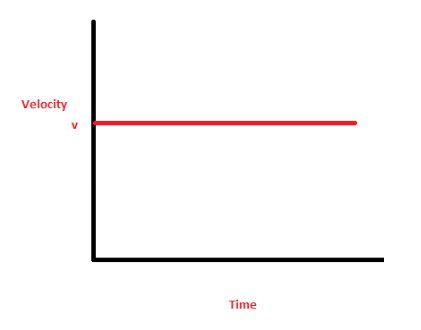# A car is moving with a constant speed of 6m/s on a straight path for one hour. Draw its Velocity - time graph

Solution:
When the velocity is constant, the velocity time graph, with Y-axis denoting velocity and X-axis denoting time, will be like:As clear from the graph, the velocity is constant (c) throughout the time interval. No particles of matter how much the time changes, the velocity will be c at every instant.

• -1
What are you looking for?